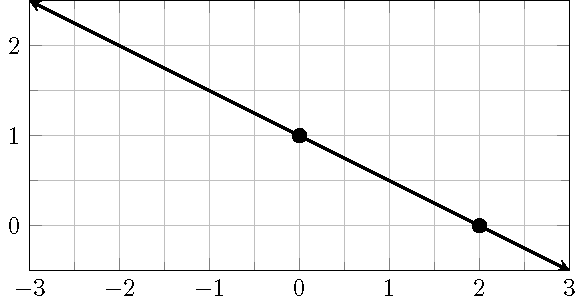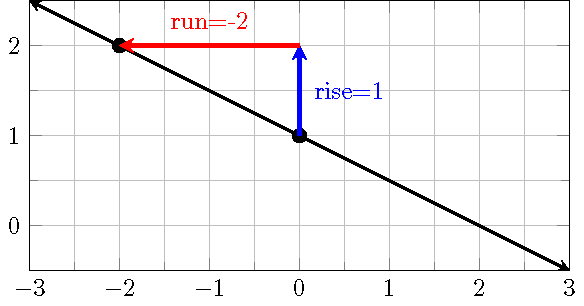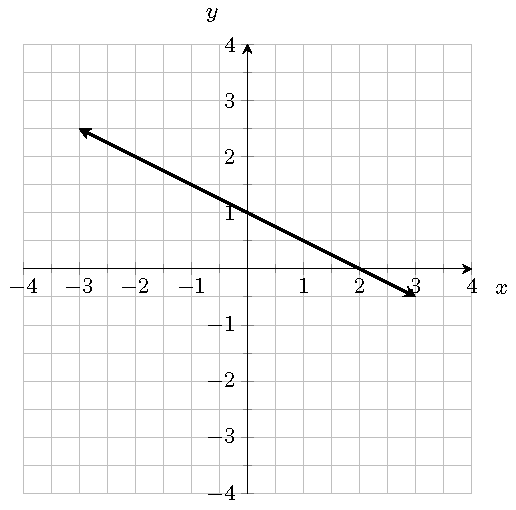# Topic 12 Linear Functions

A company has fixed costs of $10,000 for equipment and variable costs of$15 for each unit of output. The sale price for each unit is 25. What is total cost, total revenue and total profit at varying levels of output? ## 12.2 The Slope-Intercept Form Equation The slope of a line measures the steepness, in other words, “rise” over “run”, or rate of change of the line. Using the rectangular coordinate system, the slope $$m$$ of a line is defined as $m=\dfrac{y_2-y_1}{x_2-x_1}=\dfrac{\text{rise}}{\text{run}}=\dfrac{\text{change in the output }y}{\text{change in the input } x},$ where $$(x_1, y_1)$$ and $$(x_2, y_2)$$ are any two distinct points on the line. If the line intersects the $$y$$-axis at the point $$(0, b)$$, then a point $$(x, y)$$ is on the line if and only if $y=mx+b.$ This equation is called the slope-intercept form of the line. ## 12.3 Point-Slope Form Equation of a Line Suppose a line passing through the point $$(x_0, y_0)$$ has the slope $$m$$. Solving from the slope formula, we see that any point $$(x, y)$$ on the line satisfies the equation equation $y=m(x-x_0)+y_0$ which is called the point-slope form equation. ## 12.4 Linear Function A linear function $$f$$ is a function whose graph is a line. An equation for $$f$$ can be written as $f(x) = mx + b$ where $$m$$ is the slope and $$b=f(0)$$. A function $$f$$ is a linear function if the following equalities hold $\dfrac{f(x_2)-f(x_1)}{x_2-x_1} =\dfrac{f(x_3)-f(x_1)}{x_3-x_1}$ for any three distinct points $$(x_1, y_1)$$, $$(x_2, y_2)$$ and $$(x_3, y_3)$$ on the graph of $$f$$. ## 12.5 Equations of Linear Functions Example 12.1 Find the slope-intercept form equation for the linear function $$f$$ such that $$f(2)=5$$ and $$f(-1) = 2$$. Solution. 1. Find the slope $$m$$: $$m=\frac{f(2)-f(-1)}{2-(-1)}=\frac{5-2}{2-(-1)}=\frac{3}{3}=1$$. 2. Plug one of the points, say $$(2, 5)$$ in the point-slope form equation, we get $$y=1\cdot(x-2)+5$$ 3. Simplify the above equation, we get the slope-intercept form equation $$f(x)=x+3$$. ## 12.6 Graph a Linear Function by Plotting Points Example 12.2 Sketch the graph of the linear function $$f(x)=-\frac12 x + 1$$. Solution. Method 1: Get points by evaluating $$f(x)$$. 1. Choose two or more input values, e.g. $$x=0$$ and $$x=2$$. 2. Evaluate $$f(x)$$: $$f(0)=1$$ and $$f(2)=0$$. 3. Plot the points $$(0, 1)$$ and $$(2, 0)$$ and draw a line through them.Method 2: Get points by raise and run. 1. Plot the $$y$$-intercept $$(0, f(0))=(0, 1)$$. 2. Use $$\frac{\text{rise}}{\text{run}}=-\frac{1}{2}$$ to get one or more points, e.g, we will get $$(-2, 2)$$ by taking $$\text{rise}=1$$ and $$\text{run}=-2$$, i.e. move up $$1$$ unit, then move to the right $$2$$ units. 3. Plot the points $$(0, 1)$$ and $$(-2, 2)$$ and draw a line through them.## 12.7 Horizontal and Vertical Lines A horizontal line is defined by an equation $$y=b$$. The slope of a horizontal line is simply zero. A vertical line is defined by an equation $$x=a$$. The slope of a vertical line is undefined. A vertical line gives an example that a graph is not a function of $$x$$. Indeed, the vertical line test fails for a vertical line. ## 12.8 Explicit Function When studying functions, we prefer a clearly expressed function rule. For example, in $$f(x)=-\frac23x+1$$, the expression $$-\frac23x+1$$ clearly tells us how to produce outputs. For a function $$f$$ defined by an equation, for instance, $$2x+3y=3$$, to find the function rule (that is an expression), we simply solve the given equation for $$y$$. \begin{aligned} 2x+3y&=3\\ 3y&=-2x+3\\ y&=-\frac23x+1. \end{aligned} Now, we get $$f(x)=-\frac23x+1$$. ## 12.9 Perpendicular and Parallel Lines Any two vertical lines are parallel. Two non-vertical lines are parallel if and only if they have the same slope. A line that is parallel to the line $$y=mx+a$$ has an equation $$y=mx+b$$, where $$a\neq b$$. Horizontal lines are perpendicular to vertical lines. Two non-vertical lines are perpendicular if and only if the product of their slopes is $$-1$$. A line that is perpendicular to the line $$y=mx+a$$ has an equation $$y=-\frac1m x+b$$. ## 12.10 Finding Equations for Perpendicular or Parallel Lines Example 12.3 Find an equation of the line that is parallel to the line $$4x+2y=1$$ and passes through the point $$(-3, 1)$$. Solution. 1. Find the slope $$m$$ of the original line from the slope-intercept form equation by solving for $$y$$. $$y=-2x+\frac12$$. So $$m=-2$$. 2. Find the slope $$m_\parll$$ of the parallel line. $m_\parll=m=-2.$ 3. Use the point-slope form. \begin{aligned} y-1&=-2(x+3)\\ y&=-2x-5. \end{aligned} Example 12.4 Find an equation of the line that is perpendicular to the line $$4x-2y=1$$ and passes through the point $$(-2,3)$$. Solution. 1. Find the slope $$m$$ of the original line from the slope-intercept form equation by solving for $$y$$. $$y=2x-\frac12$$. So $$m=2$$. 2. Find the slope $$m_\perp$$ of the perpendicular line. $m_\perp=-\frac1m=-\frac12.$ 3. Use the point-slope form. \begin{aligned} y-3&=-\frac12(x+2)\\ y&=-\frac{1}{2}x+2. \end{aligned} ## 12.11 Practice Problem 12.1 Find the slope of the line passing through 1. $$(3,5)$$ and $$(-1, 1)$$ 2. $$(-2,4)$$ and $$(5, -2)$$. Problem 12.2 Find the point-slope form equation of the line with slope $$5$$ that passes though $$(-2, 1)$$. Problem 12.3 Find the point-slope form equation of the line passing thought $$(3, -2)$$ and $$(1,4)$$. Problem 12.4 Find the slope-intercept form equation of the line passing through $$(6, 3)$$ and $$(2, 5)$$. Problem 12.5 Determine whether the linear functions $$f(x)$$ and $$h(x)$$ with the following values $$f(-2)=-4$$ $$f(0)=h(0)=2$$ and $$h(2)=8$$ define the same function. Explain your answer. Problem 12.6 Suppose the points $$(5, -1)$$ and $$(2, 5)$$ are on the graph of a linear function $$f$$. Find $$f(-3)$$. Problem 12.7 Graph the functions. 1. $$f(x)=-x + 1$$ 2. $$f(x)=\frac{1}{2}x - 1$$ Problem 12.8 A storage rental company charges a base fee of15 and $$$x$$ per day for a small cube. Suppose the cost is$20 dollars for 10 days.

1. Write the cost $$y$$ (in dollars) as a linear function of the number of days $$x$$.
2. How much would it cost to rent a small cube for a whole summer (June, July and August)?

Problem 12.9 Find an equation for each of the following two lines which pass through the same point $$(-1, 2)$$.\

1. The vertical line.
2. The horizontal line.

Problem 12.10 Line $$L$$ is defined by the equation $$2x-5y=-3$$. What is the slope $$m_\parallel$$ of the line that is parallel to the line $$L$$? What is the slope $$m_\perp$$ of the line that is perpendicular to the line $$L$$.

Problem 12.11 Line $$L_1$$ is defined by $$3y+5x=7$$. Line $$L_2$$ passes through $$(-1, -3)$$ and $$(4, -8)$$. Determine whether $$L_1$$ and $$L_2$$ are parallel, perpendicular or neither.

Problem 12.12 Find the point-slope form and then the slope-intercept form equations of the line parallel to $$3x-y=4$$ and passing through the point $$(2,-3)$$.

Problem 12.13 Find the slope-intercept form equation of the line that is perpendicular to $$4y-2x+3=0$$ and passing through the point $$(2, -5)$$

Problem 12.14 The line $$L_1$$ is defined $$Ax+By=3$$. The line $$L_2$$ is defined by the equation $$Ax+By=2$$. The line $$L_3$$ is defined by $$Bx-Ay=1$$. Determine whether $$L_1$$, $$L_2$$ and $$L_3$$ are parallel or perpendicular to each other.

Problem 12.15 Use the graph of the line $$L$$ to answer the following questions

1. Find an equation for the line $$L$$.
2. Find an equation for the line $$L_\perp$$ perpendicular to $$L$$ and passing through $$(1,1)$$.
3. Find an equation for the line $$L_\parallel$$ parallel to $$L$$ and passing through $$(-2,-1)$$.Problem 12.16 Determine whether the points $$(-3,1)$$, $$(-2,6)$$, $$(3,5)$$ and $$(2, 0)$$ form a square. Please explain your conclusion.

Problem 12.17 A tutoring center has fixed monthly costs of $5000 that covers rent, utilities, insurance, and advertising. The center charges$60 for each private lesson and each lesson has a variable cost of \$35 to pay the instructor. Determine the number of private lessons that must be held for the tutoring center to make a profit.

Problem 12.18 In 2008, an elementary school population was 1011. By 2018 the population had grown to 1281. Assume the population changes linearly.

1. Find an equation for the population $$P$$ of the school $$t$$ years after 2008.

2. What would be the population of the school in 2021.